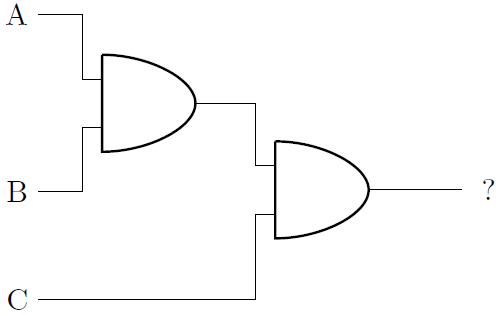# Logic problem #93432

Logic Level 1The AND gates in the diagram will output a 1 if both inputs are also 1.

If A = 1, B = 1, and C = 0, what will the final output be?

×